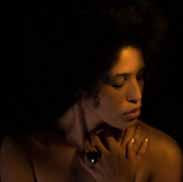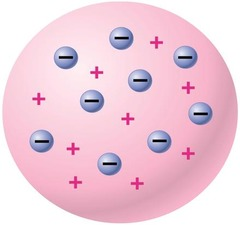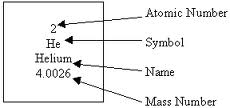# CHEMISTRY TOPIC 1: THE ATOM REVIEWJosephine Mack
question

Rutherford Experiment:

Bombarded gold foil with alpha particles. Showed atoms were mostly empty space with small, dense positively charged nucleus.
question

Bohr Model:

Small, dense, positively charged nucleus surrounded by electrons in circular orbits.
question

Wave-Mechanical Model (Modern Atomic Theory)

Small, dense, nucleus positively charged nucleus surrounded by electrons moving in \”electron cloud\”. \”Orbitals\” are areas where an electron with a certain amount of energy is most likely to be found.
question

* Each atom is made of a……*

positively charged nucleus with one or more orbiting, negatively charged electrons.
question

* Protons and neutrons are found in the *

nucleus
question

Protons have a ………..charge

positive
question

neutrons have………. charge

no
question

electrons have a………….charge

negative
question

The mass of a proton is

1 amu.
question

The mass of a neutron is

1 amu
question

The mass of an electron is almost

1/1836 amu
question

When all electrons are at their lowest possible energy, it is called the

ground state
question

When the electron gains a specific amount of energy, it moves to a higher orbital and is in the

\”excited state\”.
question

When an electron returns from a higher energy state to a lower energy state, it ……

emits a specific amount of energy usually in the form of light. This can be used to identify an element (bright line spectrum). The instrument used to see the bright line spectrum is called a spectroscope.
question

The outermost electrons are called valence electrons. These affect the…………………………

chemical properties of the element.
question

Atoms of the same element all contain the same number

of protons
question

Isotopes are atoms with…………..

equal numbers of protons but different numbers of neutrons. *different atomic masses (protons + neutrons).*
question

The average atomic mass of an element is the

weighted average of its naturally occurring isotopes.
question

JJ Thomsons modelUsed cathode ray tube Negative electrons (in mixture ) Plum pudding model !!! -uniform density
question

When an atom is neutral …

Protons = electrons
question

Mass number

Protons +neutrons
question

Mass number and atomic #question

Atomic number

# of Protons = # of electrons
question

Isotopes

Same atomic number but different mass number – different number of neutrons
question

Calculating atomic mass